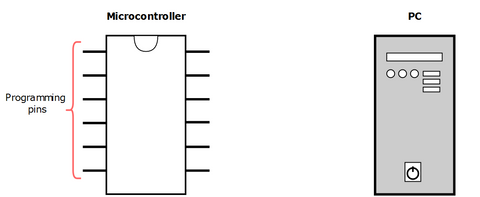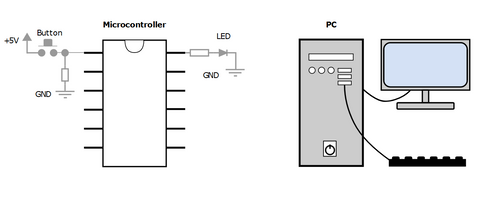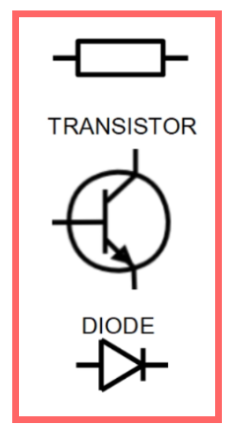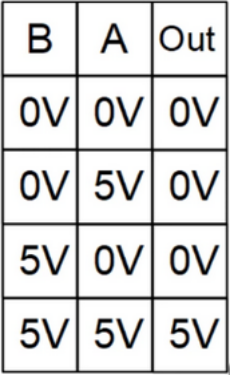# Introduction

The microcontroller is like a blank canvas, for you to implement your ideas for your gadgets or even for creating small fun games all by yourself. It also has a ton of practical uses we use everyday, maybe without even thinking about it.

A microcontroller (MCU) is a small, self-contained computer system on a single integrated circuit that is designed to control specific devices or perform specific tasks. It is a type of embedded system that contains a processor, memory, and input/output peripherals.
You can think about a microcontroller like an underpowered personal computer, as they have similar construction and structure. In a PC you have parts like CPU, memories and serial interface as separate parts whereas in a microcontroller they are all integrated in one chip.Figure 1. Microcontroller and a PC.

So just like we can connect a keyboard, headset and monitors to our PC to input and output some information we can also do that with our microcontrollers. With microcontrollers we can for example connect buttons and LED lights.

#Figure 2

We could also connect various kinds of sensors, to measure all kinds of things we might find interesting. Maybe we want to measure humidity, temperature, motion or other things, which can be done with a microcontroller. Below you’ll see a list of some of these sensors that we could apply.

• Temperature sensor
• Proximity sensor
• Infrared sensor (IR sensor)
• Ultrasonic sensor
• Light sensor
• Smoke and Gas sensor
• Alcohol sensor
• Touch sensor
• Color sensor
• Humidity sensor

So this is all really cool - all the LEDs, sensors and buttons. But how do we actually do it? We can’t just expect the microcontroller to do what we tell them to do - they wouldn’t understand. It doesn’t matter how much we scream at it. So we need to instruct the microcontroller to do what we want - the right way! So let’s figure out how to do that.

## What is a microcontroller?

What is a microcontroller and how is it made? To fully understand that, we need some basic knowledge about some of the components we find in a microcontroller. Components like the resistor, the transistor and the diode, see figure 3.Figure 3. Illustrates the symbols for a resistor, transistor and a diode.

If you aren’t quite sure what all of these components are or what they are for, we have a blog for them right here - so, if needed, check that out!

But why are these components so important for a microcontroller? With these components we can create something called logic gates, which we learned about in this blog about digital electronics.
These logic gates are very important, because they are the links that allow the data to go from analog to digital. To illustrate this, let’s go through an example with one of the gates we learned about in the blog for digital circuits. Specifically the AND gate.

In the figure below, figure 4, you’ll see the construction of a circuit of an AND gate made by the three components seen in figure 3.Figure 4. Illustrates the circuit of an AND gate.
Voltage sources: Vin, A and B
Transistors: T1 and T2.
Resistors: Ra , Rb and Rout

In the blog about analog circuits we learned that if we connect 5 V to the collector of transistor T1 (Vin) on figure 4 and there's no voltage from A or B there will be zero voltage for the output (Vout). If we change the voltage at A to 5 V there's still no voltage for the output. Same thing for B. Only when both A and B are applied 5 volts will we have 5 volts at the output, because this would mean that both transistors are open.
This concept for the circuit of the AND gate is demonstrated in the figure below.Figure 5: Notice that the only situation where we get the 5 V at the output, is when both A and B have 5 V.

If we then replace the 5 V and 0 V with 1’s and 0’s we get what is called truth tables. Remember that digital electronics only care about high and low.

When the replacement is done we have what is called a truth table.
Figure 6 is then be the truth table for the AND gate. This concept is the beginning of digital electronics and how microcontrollers understand things.Figure 6: Truth table for the AND gate.

In digital electronics there are only high and low, 1 and 0, logic levels, like the ones showcased in the truth table above. This truth table can be described using an equation. All gates have truth tables and their own equations, though the mathematics used in digital electronics is different from regular math and is called boolean algebra. This is all covered in the blog mentioned (digital circuits).

With the use of these logic gates we can then create structures that are capable of adding, multiplying or storing digital information - that are capable of working with the information. Those kind of structures are what is found inside a microcontroller, like the ADDER, MULTIPLIER and RAM.

To control and use the ADDER, MULTIPLIER and RAM we use a program. The program is stored inside the memory of the microcontroller and can be transferred through the programming pins.Figure 7: ROM stands for Read-Only Memory.

As illustrated on figure 7 can the program be thought of as a series of lines with zeros and ones. These lines could for example be as follows:Figure 8: Excerpt from figure 7.

Each line carries its own information to the memory of the microcontroller, so it knows what's going on and so it can understand our instructions. But we don’t understand the language of zeros and ones. So it would be very difficult to remember what each line of zeros and ones mean, so we have what is called an assembly language. Assembly language is for people that are really cool and good at coding and also understand the design of the specific microcontroller very well. So these generous individuals create programming languages that are easier to use, so the rest of us can program in methods that are more intuitive. They kinda connect electronics to humans in that way.

So to sum it up, when we instruct the microcontroller, the communication is translated from zeros and ones to a programming language we can understand much better. These programs could for example be C and C++, which often are used in microcontrollers.

With the help of these generous connectors we can create programs faster and easier on our microcontrollers. This is because the lines of code needed for an assembly code to produce a certain outcome is much greater for the same outcome in C and C++.

And to make it even easier for us, we can use libraries for the programming of our microcontrollers. Software libraries. A software library for a microcontroller is like a set of commands you can import into your microcontroller, to widen its tools you can program it with. It makes the microcontrollers understand certain shortcuts that are easier for us to program. You can for example import a math library so you can use different kinds of mathematical concepts in your programming. There are a ton of different libraries ready to be implemented for your next project! AND there’s a ton of very well made tutorials for microcontroller projects all over the internet, like the ones found here.

Only the imagination puts boundaries on what a microcontroller can be used for! The compact size, low power consumption, and low cost of microcontrollers make them a popular choice for many applications.

Skriv kommentar!